# Dimensional/OLAP reporting techniques with Crosstabs

I have often said that dimensional reporting is usually significantly easier than relational, the word “usual” being key. Well modeled aggregated level data from an OLAP source is easy to consume and present. The ease in which you can compare different periods, or different locations, coupled with elegant ways of handling different levels of security puts OLAP on a level above standard relational sources.

That being said, there are some limitations, and Cognos does have some difficulties in some situations. Which I will get to at some point.

For the purpose of this article we’ll take a simple crosstab, and then jazz it up a bit. In each step we’ll go over how the underlying query is affected, and a few edge cases. I am using a DMR package using DQM on Cognos 11.1.7, but the lessons here are applicable to all OLAP environments. MDX shown will be edited for the sake of clarity and brevity.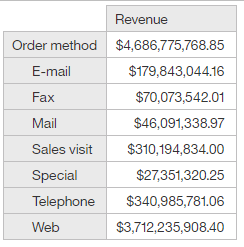We are showing revenue by order method. Notice that the first row under the header shows “Order Method”. This is the “All” member, essentially allowing the data to roll up. In this case “Order Method” is showing the sum of Revenue for each individual order method. Another way of showing the total might be to sum up all of the individual order methods using:

total(currentMeasure within set [Sales (analysis)].[Order method].[Order method].[Order method type])

Why would I prefer one way over another? Let’s take a look: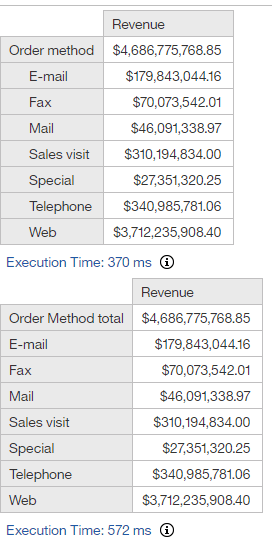The first crosstab using the All member ran slightly faster. In most OLAP sources the data is pre-aggregated at each level, so instead of having to perform a sum, it’s just pulling the value. Now let’s compare the MDX:

Crosstab 1:

```SELECT
NON EMPTY {[Measures].[Measures].[Sales (analysis)_Sales_Revenue]} ON AXIS(0),
NON EMPTY [Sales (analysis)_Order method].[Order method].MEMBERS ON AXIS(1)
FROM [go_sales]
```

Crosstab 2:

```WITH
SET XQE_NS0 AS 'HEAD([Sales (analysis)_Order method].[Order method].[Order method type].MEMBERS AS [XQE_SA0] )'
MEMBER [Sales (analysis)_Order method].[XQE_V5M_total_CM0] AS '([Sales (analysis)_Order method].[Order method].[Order method])', SOLVE_ORDER = 4
SELECT
NON EMPTY {[Measures].[Measures].[Sales (analysis)_Sales_Revenue]} ON AXIS(0),
NON EMPTY {HEAD({[Sales (analysis)_Order method].[XQE_V5M_total_CM0]}, COUNT(XQE_NS0, INCLUDEEMPTY)), [XQE_SA0]} ON AXIS(1)
FROM [go_sales]
```

The first one is fairly simple, put revenue on the columns (axis 0), and every member from the Order Method hierarchy into the rows (axis 1). The second crosstab is a little more complex. It uses with to define a set for the order methods, a calculated member for the total, and unions them together. In this case the first is ideal, but the second is perfectly reasonable if we need to limit the visible set.

Let’s take another example, finding the top 20 products: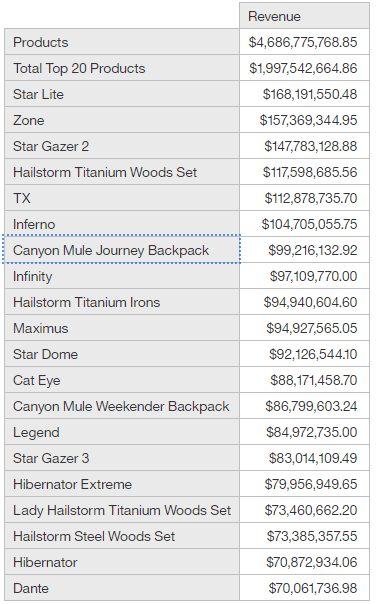Here we can see the obvious difference between the All member (Products) and the total of a set.
In the crosstab rows we have
Products: All member
Total Top 20 Products: total(currentMeasure within set [Top 20 Products])
Top 20 Products: topCount([Sales (analysis)].[Products].[Products].[Product],20,[Revenue])

Now let’s take a look at the MDX:

```WITH
SET XQE_NS0 AS 'TOPCOUNT([Sales (analysis)_Products].[Products].[Product].MEMBERS, 20, ([Measures].[Measures].[Sales (analysis)_Sales_Revenue]))'
MEMBER [Sales (analysis)_Products].[XQE_V5M_Total Top 20 Products_CM0] AS 'SUM(XQE_NS0)', SOLVE_ORDER = 4
SELECT
NON EMPTY {[Measures].[Measures].[Sales (analysis)_Sales_Revenue]} ON AXIS(0),
NON EMPTY {[Sales (analysis)_Products].[Products].[Products], HEAD({[Sales (analysis)_Products].[XQE_V5M_Total Top 20 Products_CM0]}, COUNT(XQE_NS1, INCLUDEEMPTY)), XQE_NS0} ON AXIS(1)
FROM [go_sales]
```

It’s getting a little complex here, so let’s start by explaining a few underlying concepts. When Cognos detects that nodes are peered (placed next to each other vertically in rows or horizontally in the columns), and are from the same hierarchy, it will try to turn them into a set. The All member and the top 20 are obviously from the hierarchy, but what about the Total calculation? In this case it’s turning it into a calculated member and assigning it to the hierarchy. Now it can fit nicely into the set. In MDX the curly braces {} signify a set, and the () are tuples. So we can see that axis 0 (the columns) has a measure set of just one item, Revenue. The MDX is doing something weird with the Head function, and I’m honestly not 100% certain why it’s happening, but my guess is there is some weird edge case that this covers.

Now would be a good time to talk briefly about the special OLAP objects we deal with.
Dimensions
Dimensions are essentially folders containing one or more hierarchies. They usually cover specific areas, customer hierarchies would be put into one dimension, fiscal calendar would be put into another. Cubes usually have a special dimension for measures, and right now Cognos actually only works if a cube has one.
Hierarchies
Hierarchies are the logical grouping of data within a dimension. A customer dimension might have separate hierarchies for age, gender, customer type. A calendar dimension might have a standard time hierarchy and a separate month only hierarchy.
Levels
Each hierarchy has (usually) more than one level. These are essentially the detail and rollup values for the dimensions. A calendar hierarchy might have All, Year, Quarter, Month, Day levels, while the customer type hierarchy might have All, and Type. Levels usually have names and can be selected individually, however this is not always the case. By default TM1 has unnamed levels, which can cause a great deal of consternation even to developers familiar OLAP reporting. Cubes that support Parent/Child structures will also not have named levels.
Members
Each item in a hierarchy is called a member. The years in the Year level are members, and it’s children Quarters are also members. Members from a the same hierarchy can be used in set expressions, so you could write an expression like: set(allMember, 2020, 2020 Q1, 2020 Q2, 2021). These are the bones of the query. Members are also unique in that you can use them in tuples.
Properties
Member properties are often confusing. These are extra pieces of information that provides context for each member. There are a few intrinsic properties that are common for all members, _memberUniqueName, _businessKey, _parentUniqueName, but the OLAP modeller may add as many properties as they want.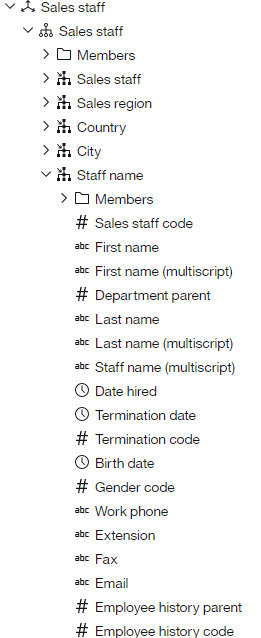Above we can see the properties for each staff member. This gives report authors plenty of options for building reports, or even filtering data. There is also nothing to stop a modeller from making a hierarchy from an item and attaching it as a property to another. In our customer example, it would be perfectly logical to have Age as both a hierarchy and as a property for the customers.
Measures
As of 11.1.7 in order for Cognos to use a cube it must have at least one measure dimension defined. This is especially frustrating since TM1, another IBM product, doesn’t actually require measure dimensions. Measures return values, which are usually numeric. Some cubes can return text, but we’ll skip that for now. Any function or calculation that requires a value can be based on a measure. If the members are the bones, measures would be the meat.
Sets
Set expressions are logical groupings of members. In an above example we used topCount to provide a set of the top performing products, but any list of members would be a set. Calling a level returns a set.
Tuples
Tuples return the intersection of specific measures and members. Trying to find a tuple of Revenue, 2019, and Tents means you want to see what the 2019 revenue was for tents. It only makes logical sense to take the items from separate hierarchies. Imagine trying to find the intersection of 2019, Knives, and 2020. 2019 and 2020 are both from the same hierarchy, so they can never have an intersection. Tuples return values.
Member Summaries
total, minimum, maximum, average, and so on. These are all functions that take the specified measure and aggregate it agains the specified set. currentMeasure is a special keyword that means take whatever measure is in context and summarize against that. Like tuples this returns a value.

Let’s take a quick look at the power that we have here. In the previous example we saw two totals, total for all products and total for only the top 20. I’m curious, how did each of the top performing products do when compared to all of the products? We could pull out a calculator and divide numbers, but let’s look for an easier way.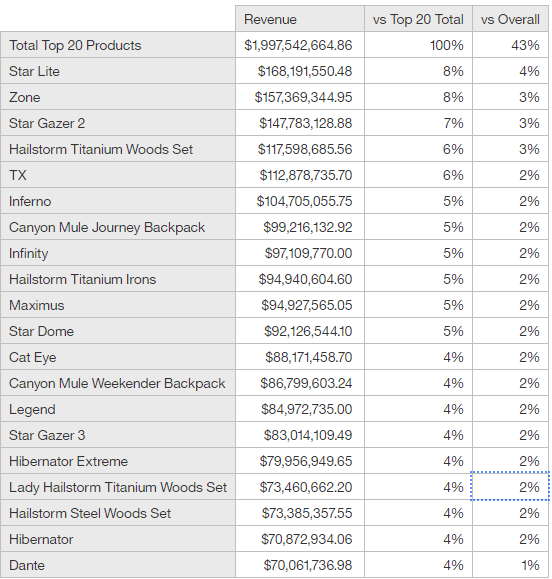There are actually a few ways to do this.
For vs Top 20 Total I’m actually ignoring the top 20 total data item. The calculation I’m using is actually percentage([Revenue] within set [Top 20 Products]) In each row it compares the revenue against the total revenue for Top 20 Products.
For vs Overall it’s a simple [Revenue] / tuple([Revenue], [Products])
Is one way better than the other? Maybe, but both methods return exactly what I would expect.

Let’s take a look at the MDX:

```WITH
SET XQE_NS0 AS 'TOPCOUNT([Sales (analysis)_Products].[Products].[Product].MEMBERS, 20, ([Measures].[Measures].[Sales (analysis)_Sales_Revenue]))'
MEMBER [Measures].[XQE_V5M_vs Top 20 Total_CM5] AS '(([Measures].[Measures].[Sales (analysis)_Sales_Revenue])/([Measures].[XQE_AG_CM1]))', SOLVE_ORDER = 4
MEMBER [Sales (analysis)_Products].[XQE_V5M_TotalTop20_CM2] AS 'SUM(XQE_NS0)', SOLVE_ORDER = 8
MEMBER [Measures].[XQE_AG_CM1] AS 'SUM(XQE_NS0, ([Measures].[Measures].[Sales (analysis)_Sales_Revenue]))', SOLVE_ORDER = 4
MEMBER [Measures].[XQE_V5M_vs Overall_CM4] AS '(([Measures].[Measures].[Sales (analysis)_Sales_Revenue])/([Measures].[Measures].[Sales (analysis)_Sales_Revenue], [Sales (analysis)_Products].[Products].[Products]))', SOLVE_ORDER = 4
SELECT
NON EMPTY {{[Measures].[Measures].[Sales (analysis)_Sales_Revenue]} AS [XQE_SA0] , HEAD({[Measures].[XQE_V5M_vs Top 20 Total_CM5]}, (COUNT(HEAD([XQE_SA0]), INCLUDEEMPTY)+COUNT(HEAD({[Measures].[XQE_V5M_vs Overall_CM4]} AS [XQE_SA1] ), INCLUDEEMPTY))), [XQE_SA1]} ON AXIS(0),
NON EMPTY {HEAD({[Sales (analysis)_Products].[XQE_V5M_TotalTop20_CM2]}, COUNT(XQE_NS1, INCLUDEEMPTY)), XQE_NS0} ON AXIS(1)
FROM [go_sales]

```

We can see that the columns is now a measure set. It’s still doing that weird thing with head(), but it’s still relatively easy to read. First we have Revenue, then we have a calculation that divides the revenue by the total of the top 20, and then a calculation that divides revenue by the tuple of revenue and the Products All member.

Something to note, in none of these queries did we reference anything from the time dimension. In this model the All member is acting as the Default Member for each dimension, but that can be defined in the model. The default member is used by the cube when nothing is referenced from a specific hierarchy. The cube modeler could say that the current year is the default, or it could even be defined based on security so the default member for the country is based on the user’s profile.

Speaking of time dimension, would this crosstab still work if we pulled in members from the time dim?This works great, but what does it to the MDX?

```WITH
SET XQE_NS1 AS 'TOPCOUNT([Sales (analysis)_Products].[Products].[Product].MEMBERS, 20, ([Measures].[Measures].[Sales (analysis)_Sales_Revenue], [Sales (analysis)_Time].[Time].DEFAULTMEMBER))'
MEMBER [Measures].[XQE_V5M_vs Top 20 Total_CM6] AS '(([Measures].[Measures].[Sales (analysis)_Sales_Revenue])/([Measures].[XQE_AG_CM3]))', SOLVE_ORDER = 4
MEMBER [Measures].[XQE_V5M_vs Top 20 Total_CM5] AS '(([Measures].[Measures].[Sales (analysis)_Sales_Revenue])/([Measures].[XQE_AG_CM3]))', SOLVE_ORDER = 4
MEMBER [Sales (analysis)_Products].[XQE_V5M_TotalTop20_CM4] AS 'SUM(TOPCOUNT([Sales (analysis)_Products].[Products].[Product].MEMBERS, 20, ([Measures].[Measures].[Sales (analysis)_Sales_Revenue])))', SOLVE_ORDER = 8
MEMBER [Measures].[XQE_AG_CM3] AS 'SUM(TOPCOUNT([Sales (analysis)_Products].[Products].[Product].MEMBERS, 20, ([Measures].[Measures].[Sales (analysis)_Sales_Revenue])), ([Measures].[Measures].[Sales (analysis)_Sales_Revenue]))', SOLVE_ORDER = 4
MEMBER [Measures].[XQE_V5M_vs Overall_CM1] AS '(([Measures].[Measures].[Sales (analysis)_Sales_Revenue])/([Measures].[Measures].[Sales (analysis)_Sales_Revenue], [Sales (analysis)_Products].[Products].[Products]))', SOLVE_ORDER = 4
MEMBER [Measures].[XQE_V5M_vs Overall_CM0] AS '(([Measures].[Measures].[Sales (analysis)_Sales_Revenue])/([Measures].[Measures].[Sales (analysis)_Sales_Revenue], [Sales (analysis)_Products].[Products].[Products]))', SOLVE_ORDER = 4
SELECT
NON EMPTY {CROSSJOIN([Sales (analysis)_Time].[Time].[Time_Time].MEMBERS, {{[Measures].[Measures].[Sales (analysis)_Sales_Revenue]} AS [XQE_SA0] , HEAD({[Measures].[XQE_V5M_vs Top 20 Total_CM5]}, (COUNT(HEAD([XQE_SA0]), INCLUDEEMPTY)+COUNT(HEAD({[Measures].[XQE_V5M_vs Overall_CM0]} AS [XQE_SA1] ), INCLUDEEMPTY))), [XQE_SA1]}), CROSSJOIN([Sales (analysis)_Time].[Time].[Year].MEMBERS, {{[Measures].[Measures].[Sales (analysis)_Sales_Revenue]} AS [XQE_SA2] , HEAD({[Measures].[XQE_V5M_vs Top 20 Total_CM6]}, (COUNT(HEAD([XQE_SA2]), INCLUDEEMPTY)+COUNT(HEAD({[Measures].[XQE_V5M_vs Overall_CM1]} AS [XQE_SA3] ), INCLUDEEMPTY))), [XQE_SA3]})} ON AXIS(0),
NON EMPTY {HEAD({[Sales (analysis)_Products].[XQE_V5M_TotalTop20_CM4]}, COUNT(XQE_NS0, INCLUDEEMPTY)), XQE_NS1} ON AXIS(1)
FROM [go_sales]

Okay, it's getting a little harder to read now, so let's focus on the interesting bit.

NON EMPTY {
CROSSJOIN(
[Sales (analysis)_Time].[Time].[Time_Time].MEMBERS,
{
{[Measures].[Measures].[Sales (analysis)_Sales_Revenue]} AS [XQE_SA0],
}
),

CROSSJOIN(
[Sales (analysis)_Time].[Time].[Year].MEMBERS,
{
{[Measures].[Measures].[Sales (analysis)_Sales_Revenue]} AS [XQE_SA2],
}
)
} ON AXIS(0),
```

It’s taking the all member from the time, and building the nested measure set below, and then doing the same thing for the years set. We can see that no matter what hierarchy we place, it should still work as expected.

Next we’ll go over properties, but first let’s talk about how text is displayed on crosstabs. If you click on a cell in the columns or rows of acrosstab and look at the pane on the right, you’ll see the “Source Type” menu. That contains the following items:
Text
Data item value
Data item label
Report Expression
Member Caption

Text is simply a text item, double click on it and add any text you want. Report expression is exactly the same as “Layout calculation”. Data item value returns, you guessed it, the value of the data item for that row. Data item label returns the label associated with that data item – mostly found in list titles. Member caption returns the caption property of any member. Items in the crosstab intersections have “Cell Value” replacing Member Caption.

An important thing to remember with crosstabs is the intersections are essentially tuples of all of the rows and columns feeding it. Every nested item can use the properties of the item of it’s parent. What does that mean? Take a look a this crosstab:The intersection there has access to details about the staff and the product. Drag in a crosstab spacer into the columns, add the properties you want into a table in the intersection. Protip: make sure to set “Define contents” on the intersection, or whatever you add to the cell will repeat in all of the fact cells.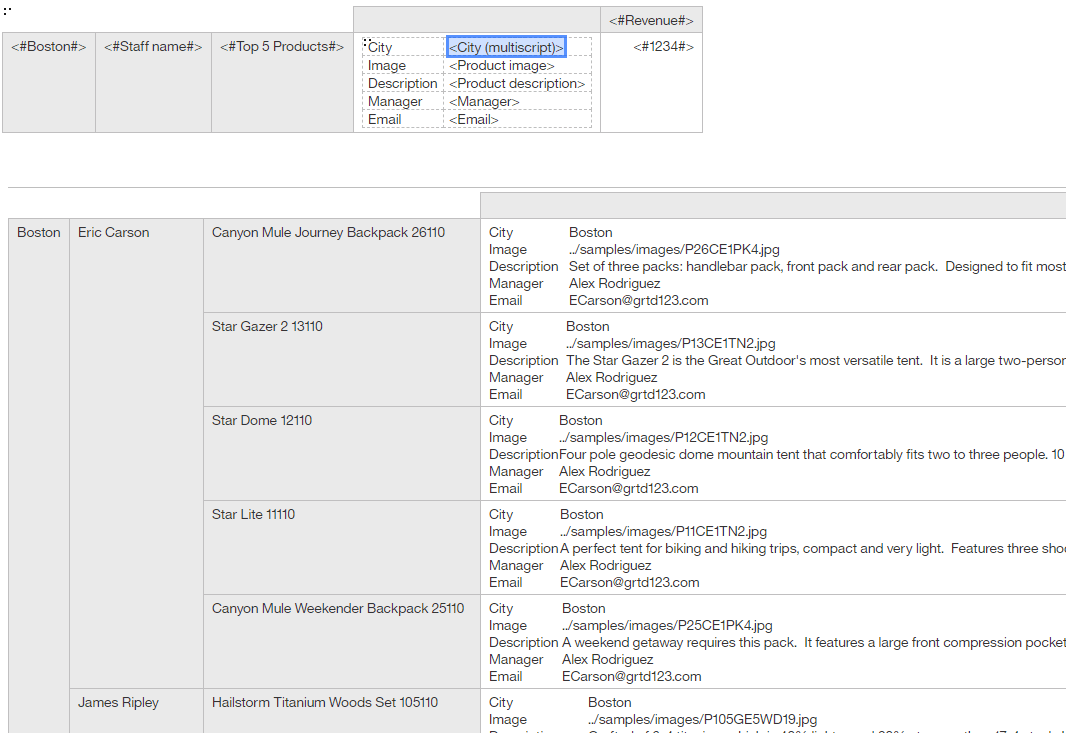A very important note, click on the “properties” row for each crossstab member node and select the appropriate items. This forces the generated MDX to include those properties in the query for that node. Forgetting to do that will result in the text items being empty.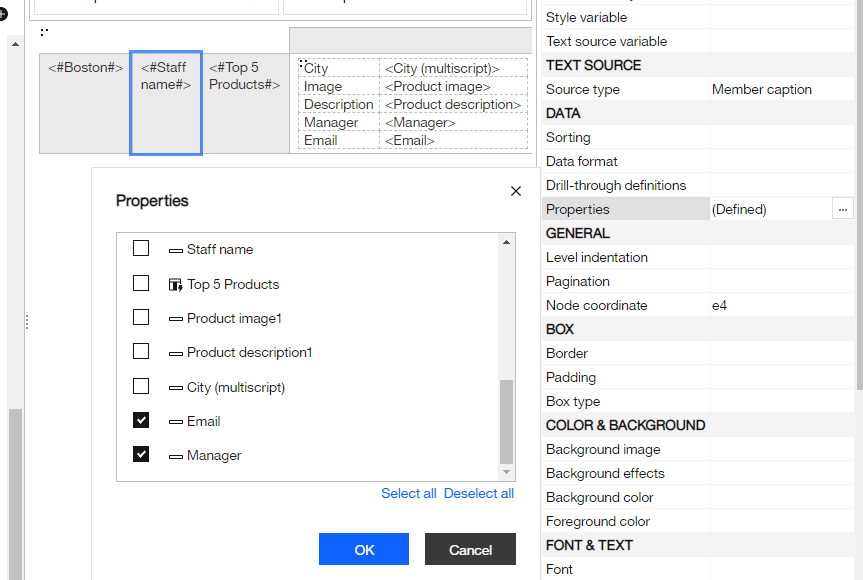Report expressions allow you to take any text value in context of the cell and use them in expressions. We could use this to use conditional styles or report variables to highlight specific rows, or even construct complex statements in the crosstab cells.

Through clever use of setting box-type none and setting define contents, it’s possible to make a crosstab look like anything.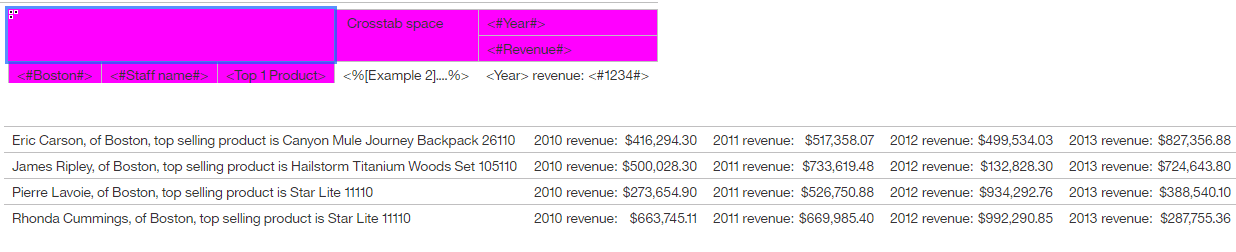Protip: One of my favorite techniques is to set the background of any hidden items to fuchsia. While some crazy people may actually want to use it in a report, I have never seen it happen. It functions as a quick and easy way to see which objects are hidden at any given time. Make sure to set visual aids to “Show Hidden Objects”, and turn off “Repeating” while you’re there.Those properties can be used in query expressions as well.

```filter([Sales (analysis)].[Sales staff].[Sales staff].[Staff name], [Sales (analysis)].[Sales staff].[Sales staff].[Staff name].[Termination date] is not null)
```

The above expression returns a set of all staff with with a termination date. Since the datatype is a date, we could also use a date prompt inside the predicate.

Once you get past the learning curve, which is admittedly steep, OLAP is an incredibly versatile datasource. Coupled with the flexibility crosstabs offer, you can do pretty much anything with them. I’m actually having trouble ending this article simply because I keep coming up with more tricks and techniques I frequently use. Attached is the report I was building to come up with the screenshots.